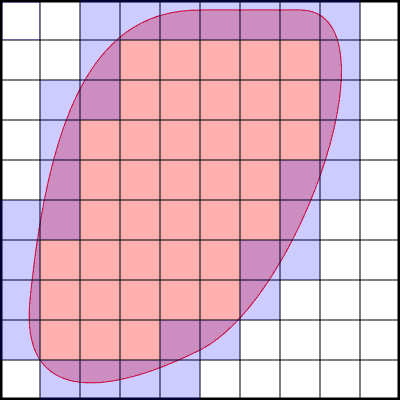# My proof of the Geometry-Real Analysis theorem

• Mike400
In summary, the conversation discusses a convex shape inside a unit square and the supremum of subsets that can be obtained from scaled and translated copies of the shape. The square is partitioned into smaller squares of three types: exterior, interior, and boundary. The conversation then introduces an equation and uses it to prove that finitely many disjoint discs can be inscribed in a unit square with a total area approaching 1. Finally, there is a question about the correctness of the proof and a request for justification for a specific step.

#### Mike400

Homework Statement
My proof of the theorem : finitely many disjoint discs can be inscribed in a unit square with total area approaching 1
Relevant Equations
$$\dfrac{i}{n^2} + \dfrac{b}{n^2} < A + \dfrac{b}{n^2} \tag1$$
$$\text{Area of finite disc packing} = e \dfrac{a- \epsilon}{n^2} + A$$
$$\left[ 1 - A - \dfrac{b}{n^2} \right] (a- \epsilon) + A<a$$
Consider a convex shape ##S## of positive area ##A## inside the unit square. Let ##a≤1## be the supremum of all subsets of the unit square that can be obtained as disjoint union of finitely many scaled and translated copies of ##S##.

Partition the square into ##n×n## smaller squares (see picture).
There are three types of such small squares: ##e## exterior squares (white in the picture), ##i## interior squares (light red in the image) and ##b## boudary squares (blue/purple). Of course ##e+b+i=n^2##$$\dfrac{i}{n^2} < A$$
$$\implies\dfrac{i}{n^2} + \dfrac{b}{n^2} < A + \dfrac{b}{n^2} \tag1$$

Picking a finite packing that covers ##\ge a-\epsilon##, for some ##\epsilon##, we can put a scaled-down copy of this packing into each of the ##e## "white" squares and, together with the original shape ##S##, obtain a finite packing of the unit square that covers ##e \dfrac{a- \epsilon}{n^2} + A##. By using this fact and equation ##(1)##

$$a>\text{Area of finite disc packing} = e \dfrac{a- \epsilon}{n^2} + A$$
$$= \dfrac{e}{n^2} (a- \epsilon) + A = \left[ 1- \left( \dfrac{i}{n^2} + \dfrac{b}{n^2} \right) \right] (a- \epsilon) + A$$
$$\geq \left[ 1- \left( A + \dfrac{b}{n^2} \right) \right] (a- \epsilon) + A$$
$$=\left[ 1 - A - \dfrac{b}{n^2} \right] (a- \epsilon) + A$$

$$\implies \left[ 1 - A - \dfrac{b}{n^2} \right] (a- \epsilon) + A<a$$

As ##n\to\infty## and ##\epsilon\to 0## the LHS converges to ##a+(1-a)A##. According to a limit theorem, this limit must be ##\le a##. Thus we conclude ##a=1##.

Thus we have shown : finitely many disjoint discs can be inscribed in a unit square with total area approaching 1.

My question : Is my proof of the theorem correct?

Last edited by a moderator:
I don't understand the last step, what happened to e for example?

Edit: I reread it and get it better now. My brain failed to string together the lines correctly.

I feel like you probably need to justify why ##b/n^2\to 0##. Other than that it seems fine.

@Mike400 pinging for visibility.

Last edited: